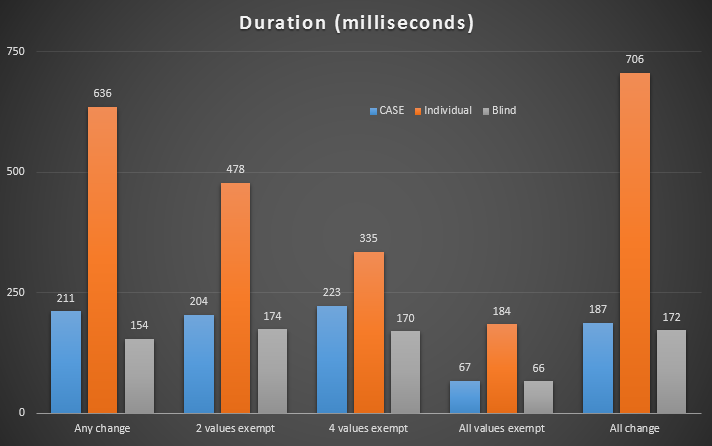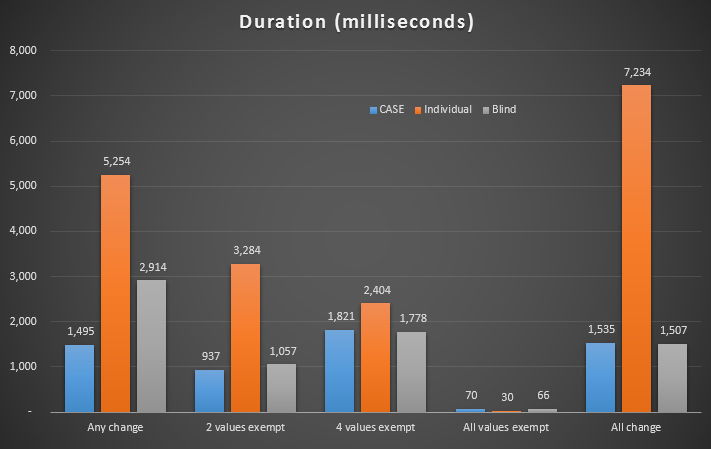# Checking if a non-LOB column needs to be updated

SentryOne eBooks

In these books, you will find useful, hand-picked articles that will help give insight into some of your most vexing performance problems. These articles were written by several of the SQL Server industry’s leading experts, including Paul White, Paul Randal, Jonathan Kehayias, Erin Stellato, Glenn Berry, Aaron Bertrand, and Joe Sack.

Featured AuthorErin Stellato is a Principal Consultant with SQLskills and a Microsoft Data Platform MVP.

Erin’s Posts

Occasionally I see people try to "optimize" their update statements to avoid writing the same value to a particular column. My understanding has always been that if you're going to update a row, assuming all of the values are in-row, the costs of locking the row are much higher than the incremental cost of updating one, two or all columns in that row.

So, I created a simple table to test this:

```CREATE TABLE dbo.whatever
(
ID INT IDENTITY(1,1) PRIMARY KEY,
v1 NVARCHAR(50) NOT NULL,
v2 NVARCHAR(50) NOT NULL,
v3 NVARCHAR(50) NOT NULL,
v4 NVARCHAR(50) NOT NULL,
v5 NVARCHAR(50) NOT NULL,
v6 NVARCHAR(50) NOT NULL
);```

Then I created a stored procedure to populate the table with 50,000 rows with a variety of small strings:

```CREATE PROCEDURE dbo.clean
AS
BEGIN
SET NOCOUNT ON;

TRUNCATE TABLE dbo.whatever;

;WITH x(d) AS
(
SELECT d FROM
(
VALUES (N'abc'),(N'def'),(N'ghi'),
(N'jkl'),(N'mno'),(N'pqr')
) AS y(d)
)
INSERT dbo.whatever(v1, v2, v3, v4, v5, v6)
SELECT TOP (50000) x1.d, x2.d, x3.d, x4.d, x5.d, x6.d
FROM x AS x1, x AS x2, x AS x3, x AS x4,
x AS x5, x AS x6, x AS x7;
END
GO```

Then I wrote update statements formulated in two ways that you could "avoid" writing to a specific column, given this variable assignment:

```DECLARE
@v1 NVARCHAR(50) = N'abc',
@v2 NVARCHAR(50) = N'def',
@v3 NVARCHAR(50) = N'ghi',
@v4 NVARCHAR(50) = N'jkl',
@v5 NVARCHAR(50) = N'mno',
@v6 NVARCHAR(50) = N'pqr';```

First by using a CASE expression to check if the value in the column is the same as the value in the variable:

```UPDATE dbo.whatever SET
v1 = CASE WHEN v1 <> @v1 THEN @v1 ELSE v1 END,
v2 = CASE WHEN v2 <> @v2 THEN @v2 ELSE v2 END,
v3 = CASE WHEN v3 <> @v3 THEN @v3 ELSE v3 END,
v4 = CASE WHEN v4 <> @v4 THEN @v4 ELSE v4 END,
v5 = CASE WHEN v5 <> @v5 THEN @v5 ELSE v5 END,
v6 = CASE WHEN v6 <> @v6 THEN @v6 ELSE v6 END
WHERE
(
v1 <> @v1 OR v2 <> @v2 OR v3 <> @v3
OR v4 <> @v4 OR v5 <> @v5 OR v6 <> @v6
);```

And second by issuing an independent UPDATE for each column (each targeting only the rows where that value had, in fact, changed):

```UPDATE dbo.whatever SET v1 = @v1 WHERE v1 <> @v1;
UPDATE dbo.whatever SET v2 = @v2 WHERE v2 <> @v2;
UPDATE dbo.whatever SET v3 = @v3 WHERE v3 <> @v3;
UPDATE dbo.whatever SET v4 = @v4 WHERE v4 <> @v4;
UPDATE dbo.whatever SET v5 = @v5 WHERE v5 <> @v5;
UPDATE dbo.whatever SET v6 = @v6 WHERE v6 <> @v6;```

Then I would compare this to the way most of us would do this today: just UPDATE all columns without caring if that was the pre-existing value for that particular column:

```UPDATE dbo.whatever SET
v1 = @v1, v2 = @v2, v3 = @v3,
v4 = @v4, v5 = @v5, v6 = @v6
WHERE
(
v1 <> @v1 OR v2 <> @v2 OR v3 <> @v3
OR v4 <> @v4 OR v5 <> @v5 OR v6 <> @v6
);```

(These all assume that the columns and the parameters/variables are not NULLable – they would need to use COALESCE to account for comparing NULLs on either side if that is the case. They also assume you would have an additional WHERE clause to target specific rows – in this example you could run the first and third queries without the all-encompassing WHERE clause and see nearly identical results. I kept this simple for brevity.)

Then I wanted to see what happens in these three cases when any value might be changed, when particular values might be changed, when no values would be changed, and when all values will be changed. I could affect this by changing the stored procedure to insert constants into particular columns, or by changing the way variables were assigned.

```-- to show when any value might change in a row, the procedure uses the full cross join:

SELECT TOP (50000) x1.d, x2.d, x3.d, x4.d, x5.d, x6.d

-- to show when particular values will change on many rows, we can hard-code constants:

-- two values exempt:
SELECT TOP (50000) N'abc', N'def', x3.d, x4.d, x5.d, x6.d

-- four values exempt:
SELECT TOP (50000) N'abc', N'def', N'ghi', N'jkl', x5.d, x6.d

-- to show when no values will change, we hard-code all six values:

SELECT TOP (50000) N'abc', N'def', N'ghi', N'jkl', N'mno', N'pqr'

-- and to show when all values will change, a different variable assignment would take place:

DECLARE
@v1 NVARCHAR(50) = N'zzz',
@v2 NVARCHAR(50) = N'zzz',
@v3 NVARCHAR(50) = N'zzz',
@v4 NVARCHAR(50) = N'zzz',
@v5 NVARCHAR(50) = N'zzz',
@v6 NVARCHAR(50) = N'zzz';
```

## Results

After running these tests, the "blind update" won in every single scenario. Now, you're thinking, what's a couple hundred milliseconds? Extrapolate. If you're performing a lot of updates in your system, this can really start to take a toll.Detailed results in Plan Explorer: Any change | 2 values exempt | 4 values exempt | All values exempt | All change

Based on feedback from Roji, I decided to test this with a few indexes as well:

```CREATE INDEX x1 ON dbo.whatever(v1);
CREATE INDEX x2 ON dbo.whatever(v2);
CREATE INDEX x3 ON dbo.whatever(v3) INCLUDE(v4,v5,v6);```

Durations were substantially increased with these indexes:Detailed results in Plan Explorer: Any change | 2 values exempt | 4 values exempt | All values exempt | All change

## Conclusion

From this test, it seems to me that it is usually not worth checking if a value should be updated. If your UPDATE statement affects multiple columns, it is almost always cheaper for you to scan all of the columns where any value might have changed rather than check each column individually. In a future post, I will investigate whether this scenario is paralleled for LOB columns.